##Dividend Regressions: January 2023

Variables used in the regression

1. Dividend Yield = Dividends per share in most recent year/ Current Stock Price
2. Dividend Payout Ratio = Dividends / Net Income
3. Beta: Regression or Bottom up beta
4. Expected Growth in EPS over next 5 years = Consensus analyst estimate (or your own) of expected growth in EPS . If you don't have an analyst estimate, use your own estimate of expected growth.

US Regression: Dividend Yield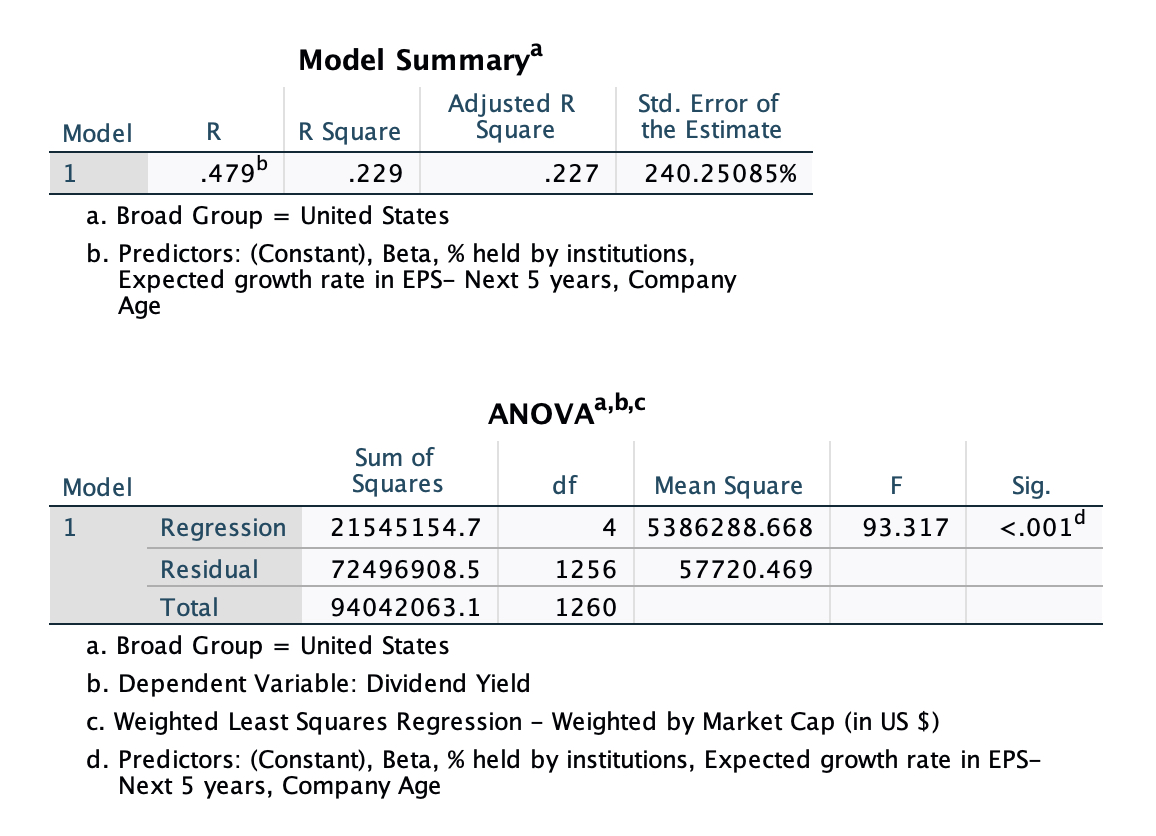### Regression Output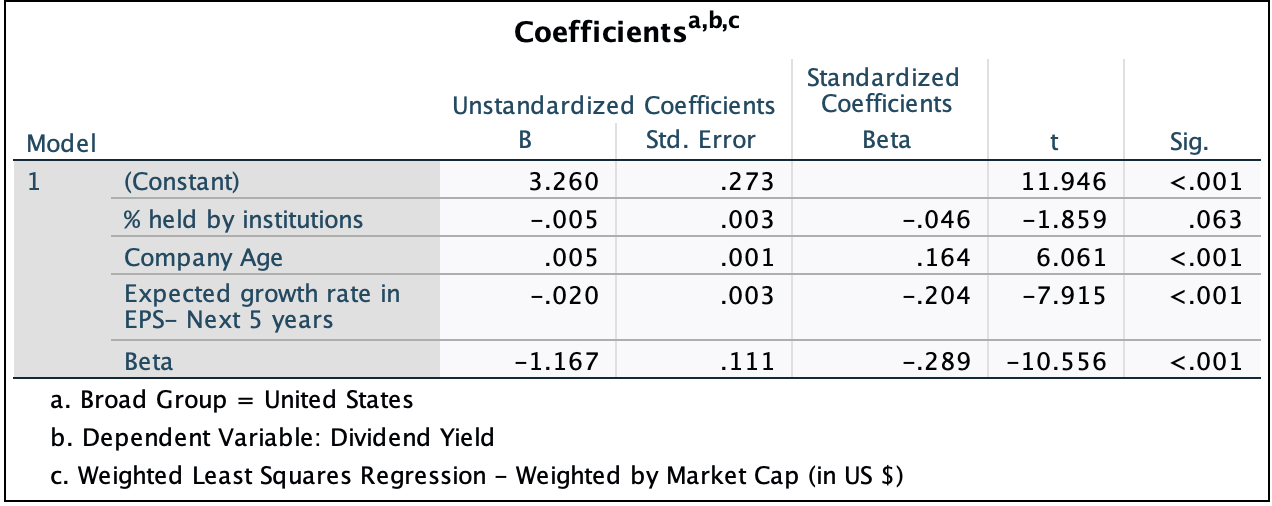US Regression: Dividend Payout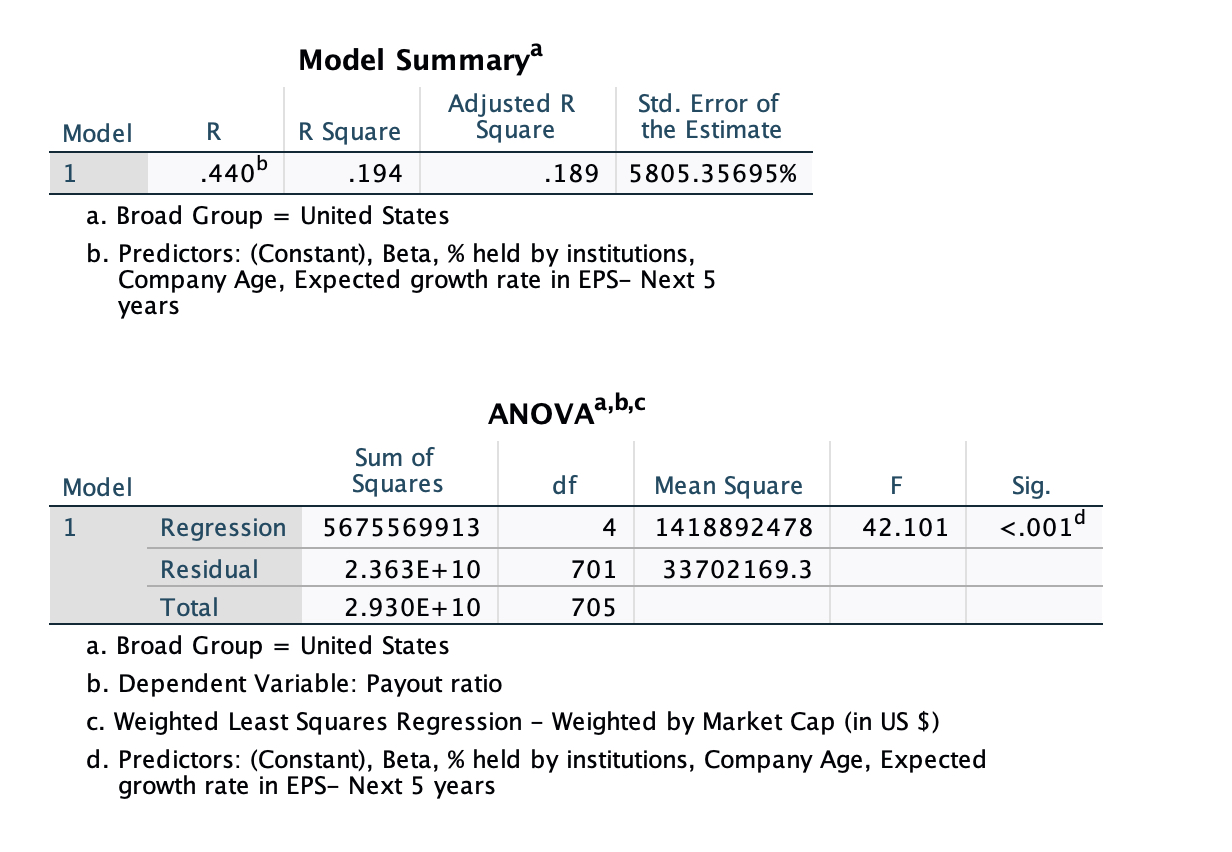### Regression Output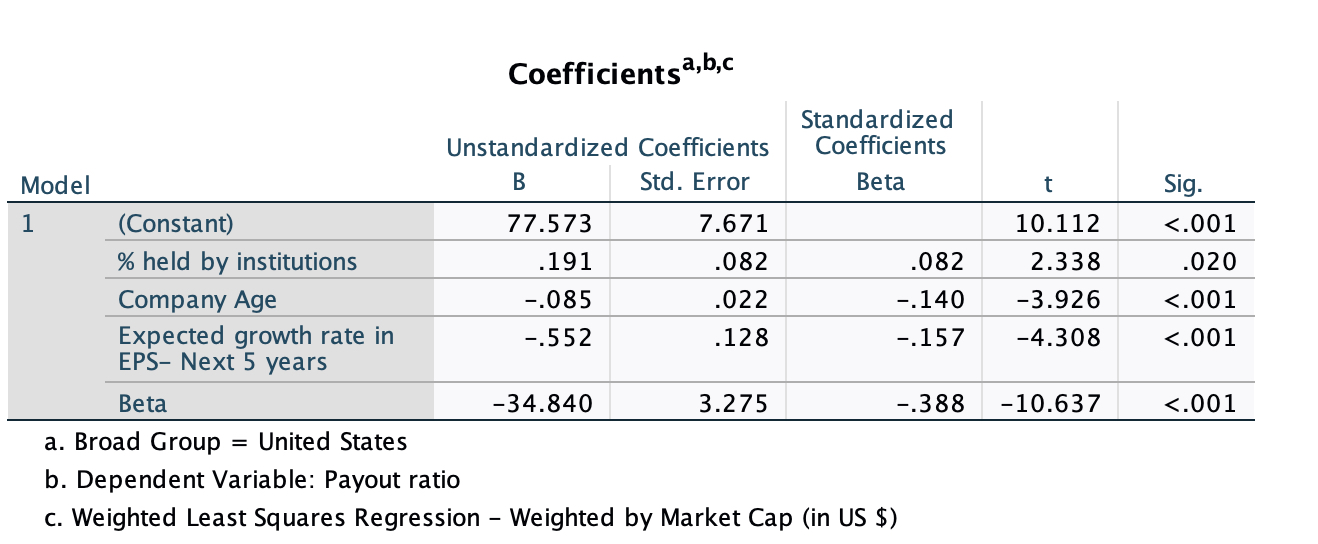Global Regression: Dividend Yield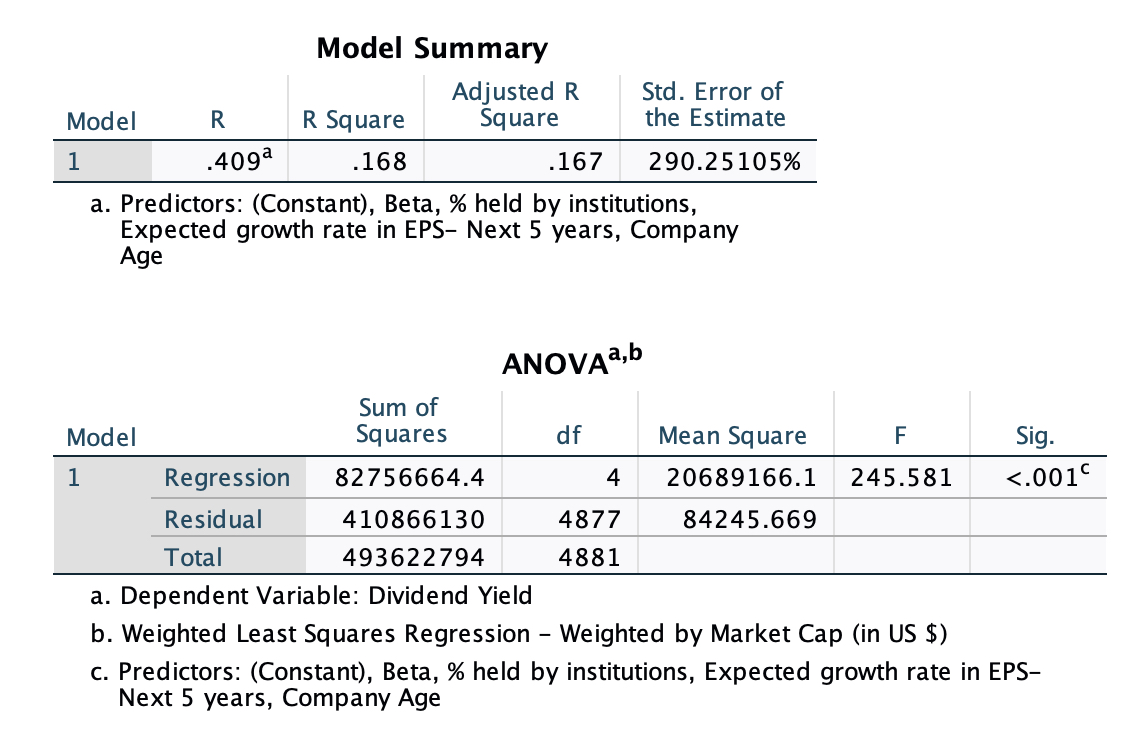### Regression Output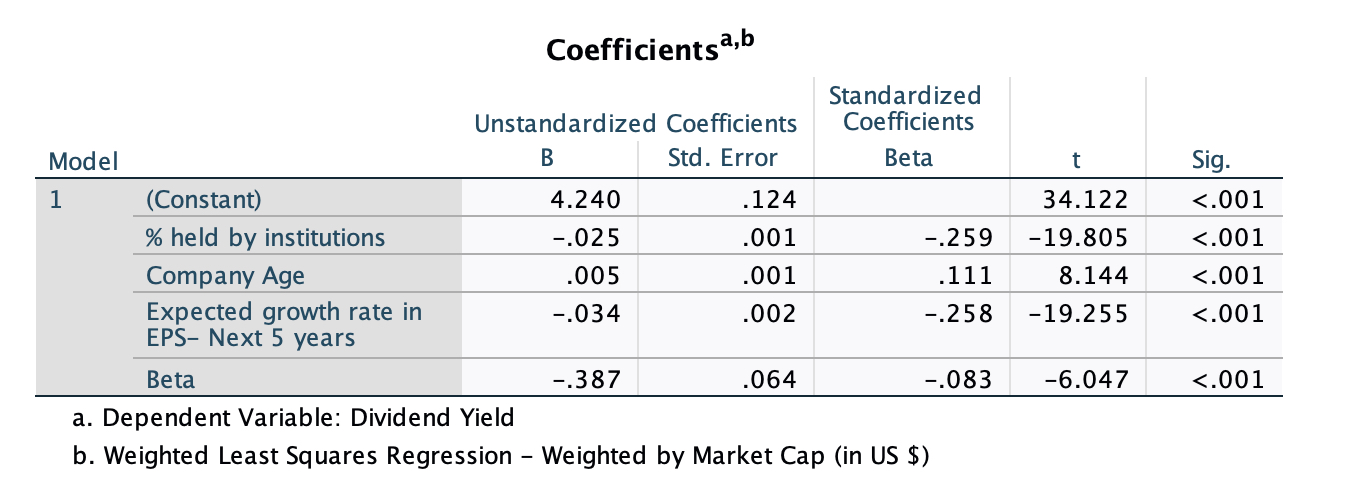Global Regression: Dividend Payout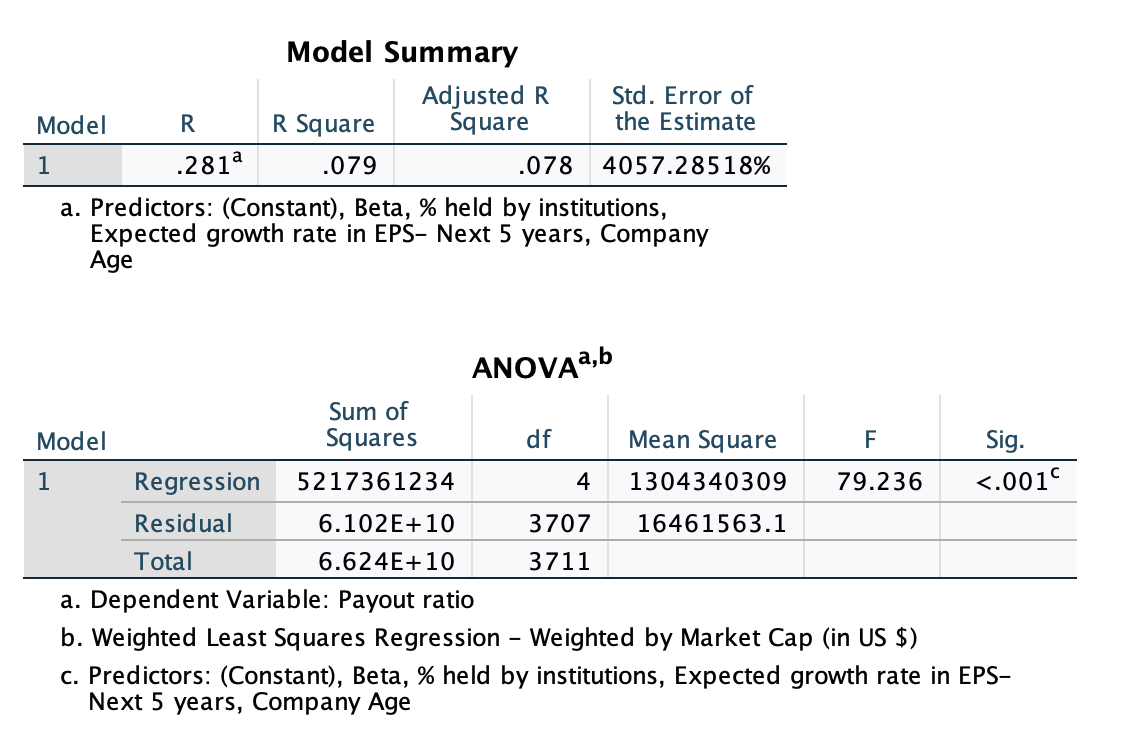### Regression Output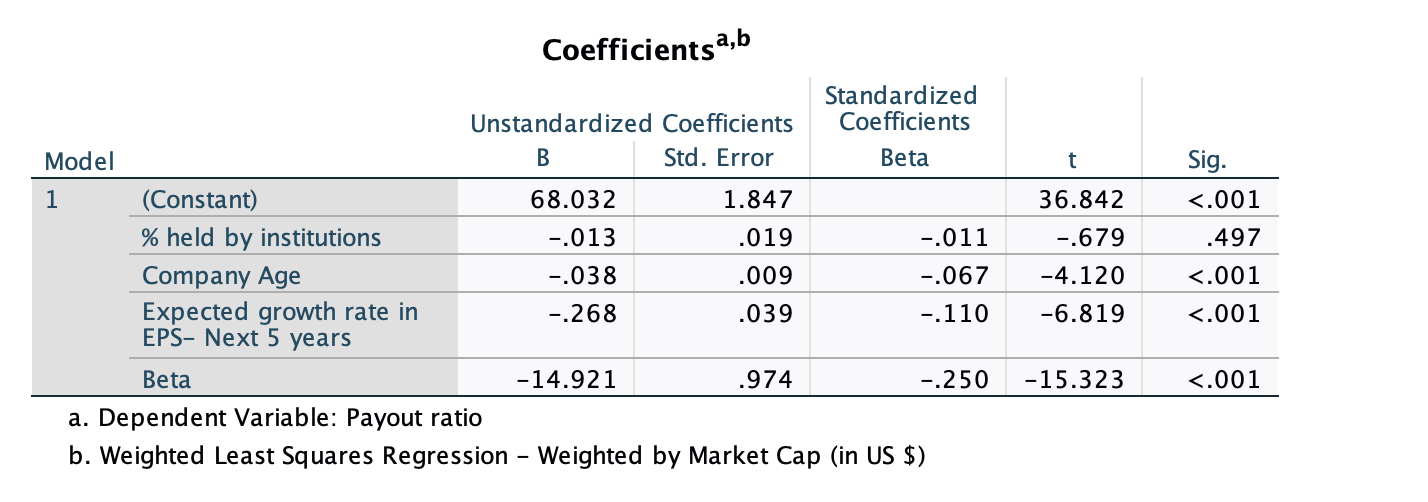• How do I use this regression?

Assume that you want to estimate the dividend payout ratio for a firm with the following characteristics, using the US regression:

% held by instiutions = 30%

Regression beta = 1.20

Expected Growth in EPS over next 5 years = 10%

Company Age = 35

• Predicted Values
• Expected Dividend Yield = 3.26 - .005 (30) + 0.005 (35) - 0.020 (10) - 1.167 (1.20) = 1.6846 or 1.68%
• Expected Dividend payout ratio = 77.57 + 0.191 (30) - 0.085(35) + 0.552 (10) -34.84 (1.20) = 44.04 or 44.04%

If your predicted value is less than zero, your predicted dividend payout ratio is zero.